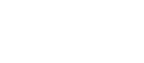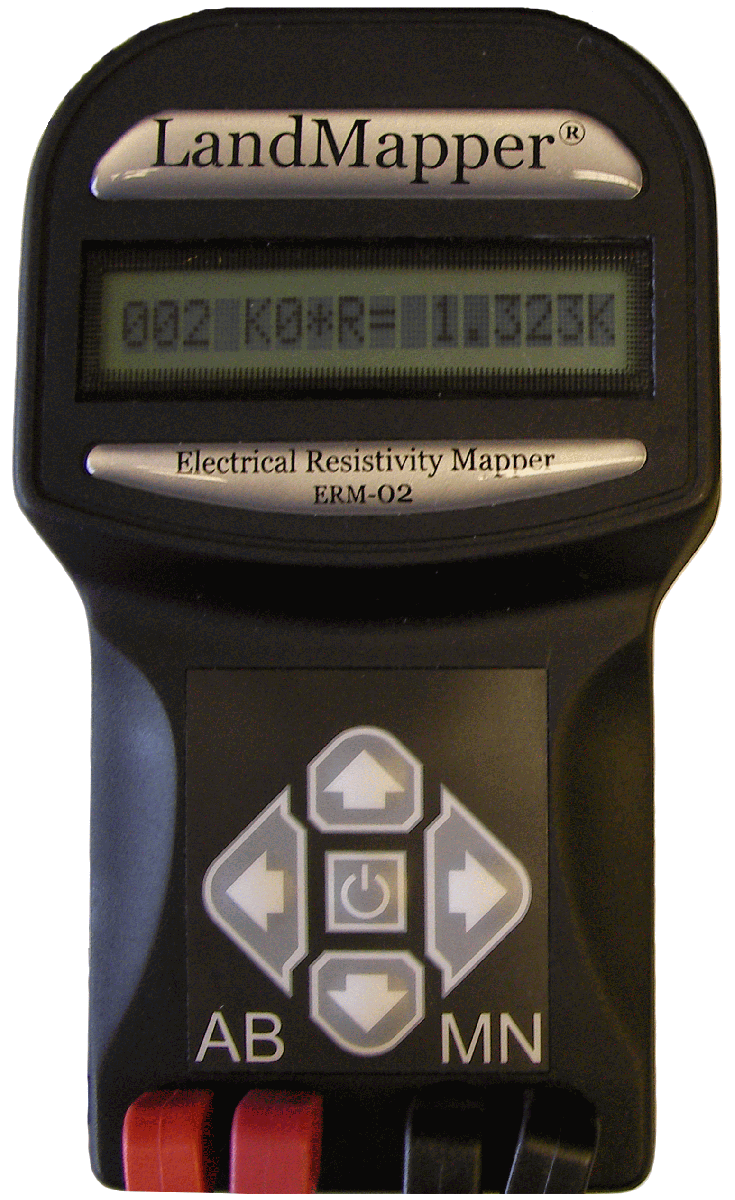Landviser, LLC Enlightening Research
 Search this site:

# Setting K coefficient in LandMapper memory

##LandMapper ERM-02 is usually supplied with one four-electrode probe in Wenner configuration (optional) and the probe-specific coefficient K is preset in the device memory (K1). If you ordered or build multiple probes/cable arrays, you can change K1-K9 coefficients in the device. Note: K0=1 always and is not changeable by user!

1. Press the power buttonto turn the device on. As the device is turned on the brief message "ASTRO-LANDVISER" is displayed on the screen of the device.
2. Press and hold the FUNCTION key (►) and press the UP key (▲) to enter the coefficient changing mode.
3. Scroll through K coefficients with the keys (▲) or (▼).  Change the value of the coefficient with the keys (◄) and (▲) or (▼). The digit to be changed is selected with the INPUT key (◄), the digit starts blinking and can be changed with the keys (▲) or (▼). The value of K0=01.00 is constant and cannot be changed by the user. If a measurement is taken using K0, the resulting output is resistance, not resistivity, and can be useful when the geometry of the array is constantly changing, as in 2D imaging or electrical tomography. In this case the resistance values can be multiplied by corresponding K coefficients according to the arrays used to obtain the resistivity. In conductivity mode, when measurements are taken with K0, the measured value is conductance, not conductivity, and the results can be converted to conductivity after measurements with appropriate geometrical coefficient.

Note: User only needs to calculate and enter the K-coefficients of the four-electrode probes for resistivity mode, the device automatically outputs results as resistivity or conductivity depending on mode selected and recalculates coefficient accordingly.

 Conductivity (µS/cm) Resistivity (Ω-cm) Dissolved Solids (ppm) .056 18,000,000 .0277 .084 12,000,000 0.417 .167 6,000,000 0.833 1.00 1,000,000 .500 2.50 400,000 1.25 20.0 50,000 10.0 200 5000 100 2000 500 1,000 20,000 50 10,000
1. To store the new value of the K coefficient press and hold the (►) key, then press the (◄) key. The new value of K coefficient is stored in the device’s memory and the value is not blinking.

Display listing:

KN = --,--

KN is an ID of the K coefficient (from K0 to K9)

--, -- is the value of the K coefficient from 00.01 up to 99.99

Note: K coefficients depend on probe/cell size. You can build probes suitable to different media.  Solutions/soils with an extremely high conductivity require a sensor with a probe constant greater than 1.0. Solutions/soils with extremely low conductivity require a sensor with a probe constant less than 1.0. The greater the distance between the electrodes, the smaller the current signal (www.omega.com).